Explore BrainMass

# Declining-Balance Method of Depreciation

Declining balance depreciation is often called accelerated depreciation. This method of depreciation allows a company to charge a disproportionate amount of depreciation expense against revenue in the first years of an asset’s life. The justification for this method is that the usefulness of a new asset is usually greater in its early life than in its later years.

Calculating Declining-Balance Depreciation

Step 1: Calculate the assets carrying amount or net book value. The asset’s carrying amount is equal to the amount that the asset’s historical cost exceeds its accumulated depreciation. You’ll notice that salvage value is not needed in this calculation, unlike other methods of depreciation.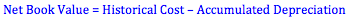Step 2: Find the depreciation rate. Accountants usually decide to base the declining-balance depreciation rate on some multiple of the straight-line depreciation rate. The straight-line rate is found by dividing the value “1” by the asset’s useful life in years.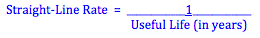The most popular method is to use double the straight-line rate. This is called ‘double-declining balance method’. Under this method, the multiple we use is “2” or 200%.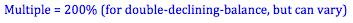The depreciation rate is found by multiplying the “multiple” by the straight-line rate.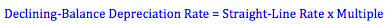Step 3: Find the depreciation expense. The actual depreciation expense is found by multiplying the asset’s net book value by the declining-balance depreciation rate.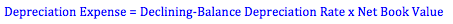It is important to note that the depreciable amount when using an accelerated method is based on the net book value of the asset. As accumulated depreciation increases, the net book value decreases. As a result, the depreciation rate will apply to a lower book value each subsequent year. This is why more depreciation expense is recognized in earlier periods rather than later period.

Step 4: Record depreciation. To record depreciation, we debit an expense account for depreciation for the period and credit the contra-asset accumulated depreciation. The depreciation expense account is closed each year during the closing entries. The accumulated depreciation account is a permanent account that equals the value of all the depreciation expense recognized to date.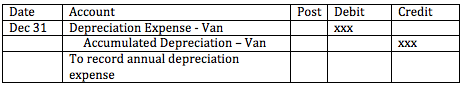When other methods of depreciation are used, the same accounts are debited and credited, but the amounts may be different.